﻿ 热电联产在集中供热改造中的节能分析 Energy Saving Analysis of CHP in the Heat Supply Modification

Advances in Energy and Power Engineering
Vol.06 No.01(2018), Article ID:23726,19 pages
10.12677/AEPE.2018.61004

Energy Saving Analysis of CHP in the Heat Supply Modification

Hongbin Zhao1,2, Xuan Hou1,2, Jiawang Li1,2

1College of Machinery and Transportation Engineering, China University of Petroleum, Beijing

2Beijing Key Laboratory of Process Fluid Filtration and Separation, Beijing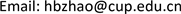Received: Jan. 17th, 2018; accepted: Feb. 2nd, 2018; published: Feb. 11th, 2018ABSTRACT

Taking the reconstruction project of one central heating system as an example, this paper puts forward the transformation scheme of the combined heat and electricity generation, and analyzes the energy consumption of the transformed heating system. The total coal consumption of power generation and heating was saved 513,386 t per year after energy saving transformation. The exergy efficiency of the original heating boiler is 15.35%, after transforming, it’s 42.6%. While through exergy analysis, it is found that the boiler’ exergy loss rate is the maximum, accounting for 81.2% of the system’s total exergy loss. Additionally, the thermal performance of thermal power plant is analyzed, and the total efficiency of thermal power plant can be improved obviously by increasing the amount of air supply. Thus it can be seen that this reform scheme can effectively improve system efficiency and has certain promotion value.

Keywords:CHP (Combined Heat and Power), Central Heating, Analysis of Energy Consumption

1中国石油大学(北京)机械与储运工程学院，北京

2过程流体过滤与分离技术北京市重点实验室，北京1. 引言

2. 方案概述Table 1. Statistics table for original source of heatTable 2. Statistics table for improved source of heat

3. 模型建立

3.1. 热电联产的能耗计算模型

Bld——生产电能的年消耗燃料量；Blr——供应热能的年消耗燃料量；Q0——热电联产总耗热量；Qc——抽汽供热量；Q——热用户所需热量； ${{W}^{\prime }}_{d}$ ——热电厂年产电量。

${Q}_{0}={D}_{0}\left({h}_{0}-{{h}^{\prime }}_{0}\right)+{D}_{zr}×\delta$ (1)

${Q}_{r}={D}_{r}×\left({h}_{r}-{{h}^{\prime }}_{r}\right)$ (2)

${Q}_{d}={Q}_{0}-{Q}_{r}$ (3)

${q}_{d}=\frac{{Q}_{d}}{{P}_{e}}$ (4)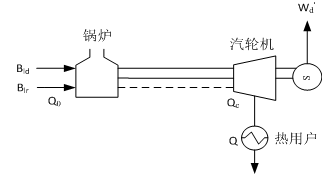Figure 1. The energy balance of CHP

${b}_{ld}=\frac{{Q}_{d}}{{P}_{e}×29271}$ (5)

${B}_{ld}={b}_{ld}×{{W}^{\prime }}_{d}$ (6)

${b}_{lr}=\frac{{10}^{6}}{29271{\eta }_{lr}}=\frac{34.2}{{\eta }_{lr}}$ (7)

${B}_{lr}={b}_{lr}×{Q}_{nr}$ (8)

${B}_{l}={B}_{ld}+{B}_{lr}$ (9)

3.2. 热电厂的热力性能计算模型

3.2.1. 热电厂总效率

${\eta }_{z}=\frac{3600{W}_{z}+{Q}_{r}}{{Q}_{br}}$ (10)

Qbr可按下式计算：

${Q}_{br}=29271×B$ (11)

3.2.2. 热电比

$\theta =\frac{{Q}_{r}}{3600×{W}_{z}}$ (12)

3.2.3. 发电热效率

${\eta }_{fd}=\frac{3600×{W}_{z}}{{Q}_{br}}$ (13)

3.2.4. 供热效率

${\eta }_{gr}=\frac{{Q}_{r}}{{Q}_{br}}$ (14)

3.3. 热电联产的㶲分析计算模型

3.3.1. 锅炉的㶲损失计算

${E}_{rl}+{E}_{k}={E}_{yx}+{I}_{g}$ (15)

${E}_{yx}={D}_{m0}{e}_{b}-{D}_{fw}{e}_{fw}+{D}_{mr}\left({e}_{rc}-{e}_{rj}\right)$ (16)

${I}_{g}={E}_{rl}+{E}_{k}-{E}_{yx}$ (17)

${\eta }_{eb}=\frac{{E}_{yx}}{{E}_{rl}}×100%$ (18)

3.3.2. 汽轮机的㶲损失计算

${D}_{m0}{e}_{q}+{D}_{mr}{e}_{rz}-{D}_{mg}{e}_{rg}=\sum _{i=1}^{7}{D}_{i}{e}_{i}+{D}_{n}{e}_{n}+{D}_{c}{e}_{c}+{W}_{t}+{I}_{qj}$ (19)

${I}_{qj}={D}_{m0}{e}_{q}+{D}_{mr}{e}_{rz}-{D}_{mg}{e}_{rg}-\sum _{i=1}^{7}{D}_{i}{e}_{i}-{D}_{c}{e}_{c}-{D}_{n}{e}_{n}-{W}_{t}$ (20)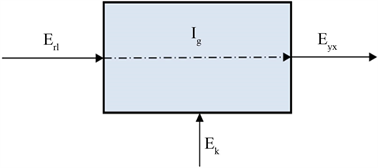Figure 2. Black-box model of boiler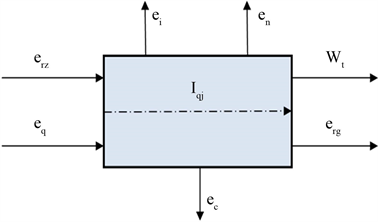Figure 3. Black-box model of steam turbine

${\eta }_{et}=\frac{{W}_{t}+{D}_{c}{e}_{c}}{{D}_{m0}{e}_{q}+{D}_{mr}{e}_{rz}-{D}_{mg}{e}_{rg}-\sum _{i=1}^{7}{D}_{i}{e}_{i}-{D}_{n}{e}_{n}}×100%$ (21)

3.3.3. 高压加热器的㶲损失计算

1号高压加热器的㶲平衡方程为：

${D}_{1}{e}_{1}={D}_{fw}\left({e}_{w1}-{e}_{w2}\right)+{D}_{1}{e}_{d1}+{I}_{g1}$ (22)

2号高压加热器的㶲平衡方程为：

${D}_{2}{e}_{2}+{D}_{1}{e}_{d1}={D}_{fw}\left({e}_{w2}-{e}_{w3}\right)+\left({D}_{1}+{D}_{2}\right){e}_{d2}+{I}_{g2}$ (23)

3号高压加热器的㶲平衡方程为：

${D}_{3}{e}_{3}+\left({D}_{1}+{D}_{2}\right){e}_{d2}={D}_{fw}\left({e}_{w3}-{e}_{w4}\right)+\left({D}_{1}+{D}_{2}+{D}_{3}\right){e}_{d3}+{I}_{g3}$ (24)

${I}_{gj}={I}_{g1}+{I}_{g2}+{I}_{g3}$ (25)

${\eta }_{eh}=\frac{{D}_{fw}\left({e}_{w1}-{e}_{w4}\right)}{\sum _{i=1}^{3}{D}_{i}{e}_{i}-{e}_{d3}\sum _{i=1}^{3}{D}_{i}}×100%$ (26)

3.3.4. 除氧器的㶲损失计算

${D}_{4}{e}_{4}+{e}_{d3}\sum _{i=1}^{3}{D}_{i}={D}_{fw}{e}_{w4}-{D}_{cw}{e}_{w5}+{I}_{cy}$ (27)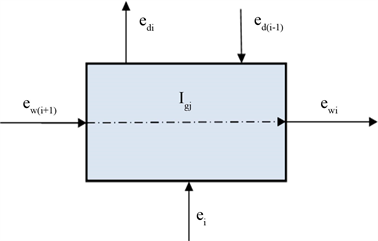Figure 4. Black-box model of high pressure heater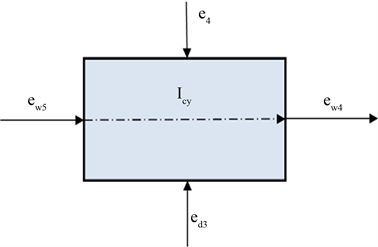Figure 5. Black-box model of deaerator

${I}_{cy}={D}_{4}{e}_{4}+{e}_{d3}\sum _{i=1}^{3}{D}_{i}+{D}_{cw}{e}_{w5}-{D}_{fw}{e}_{w4}$ (28)

${\eta }_{ec}=\frac{{D}_{fw}{e}_{w4}-{D}_{cw}{e}_{5}}{{D}_{4}{e}_{4}-{e}_{d3}\sum _{i=1}^{3}{D}_{i}}×100%$ (29)

3.3.5. 低压加热器的㶲损失计算

5号低压加热器的㶲平衡方程为：

${D}_{5}{e}_{5}={D}_{cw}\left({e}_{w5}-{e}_{w6}\right)+{D}_{5}{e}_{d5}+{I}_{d5}$ (30)

6号高压加热器的㶲平衡方程为：

${D}_{6}{e}_{6}+{D}_{5}{e}_{d5}={D}_{cw}\left({e}_{w6}-{e}_{w7}\right)+\left({D}_{5}+{D}_{6}\right){e}_{d6}+{I}_{d6}$ (31)

7号高压加热器的㶲平衡方程为：

${D}_{7}{e}_{7}+\left({D}_{5}+{D}_{6}\right){e}_{d6}={D}_{cw}\left({e}_{w7}-{e}_{w8}\right)+\left({D}_{5}+{D}_{6}+{D}_{7}\right){e}_{d7}+{I}_{d7}$ (32)

${I}_{dj}={I}_{d5}+{I}_{d6}+{I}_{d7}$ (33)

${\eta }_{ei}=\frac{{D}_{cw}\left({e}_{w5}-{e}_{w8}\right)}{\sum _{i=5}^{7}{D}_{i}{e}_{i}-{e}_{d7}\sum _{i=5}^{7}{D}_{i}}×100%$ (34)

3.3.6. 凝汽器的㶲损失计算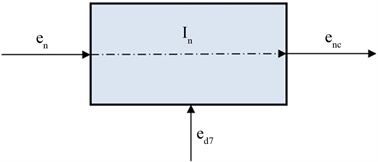Figure 6. Black-box model of condenser

${D}_{n}{e}_{n}+{e}_{d7}\sum _{i=5}^{7}{D}_{i}={D}_{cw}{e}_{nc}+{I}_{n}$ (35)

${I}_{n}={D}_{n}{e}_{n}+{e}_{d7}\sum _{i=5}^{7}{D}_{i}-{D}_{cw}{e}_{nc}$ (36)

3.3.7. 热网的㶲损失计算

${I}_{rw}={D}_{c}\left({e}_{c}-{e}_{r}\right)$ (37)

3.3.8. 各设备㶲损率的计算

㶲损率是各个设备的㶲损失与系统总㶲损失的比值，计算公式如下：

${e}_{li}=\frac{{I}_{i}}{{I}_{qjz}}$ (38)

${I}_{qjz}={I}_{g}+{I}_{qj}+{I}_{gj}+{I}_{cy}+{I}_{dj}+{I}_{n}+{I}_{rw}$ (39)

4. 计算结果及能耗分析

4.1. 热电联产与热电分产的能耗对比Table 3. Coal consumption of electric energy in condensing power plantTable 4. Coal consumption of heat energy in district boilersTable 5. Coal consumption of electric energy in CHPTable 6. Coal consumption of heat energy in thermal power plantTable 7. Coal consumption of heat energy in reserved district boilersTable 8. Comparison of Coal consumption in two different methods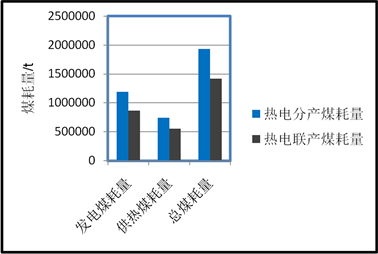Figure 7. Black-box model of condenser

4.2. 热电厂的热力性能分析

4.2.1. 热电厂技术指标随抽汽量的变化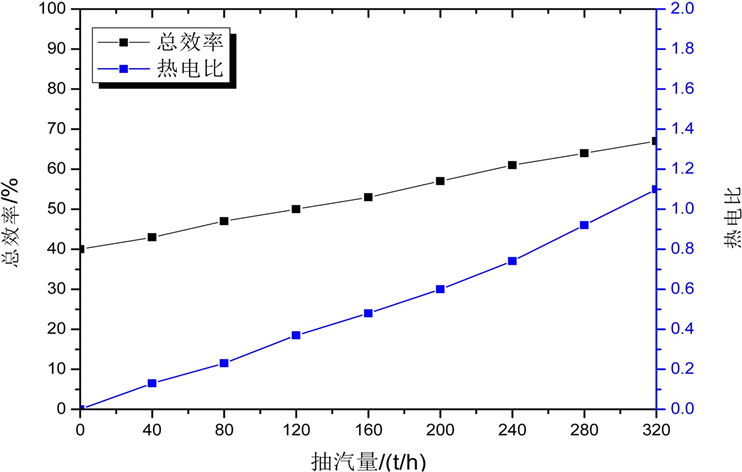Figure 8. The change of overall efficiency and ratio of heat to electricityFigure 9. the change of generating efficiency and heating efficiency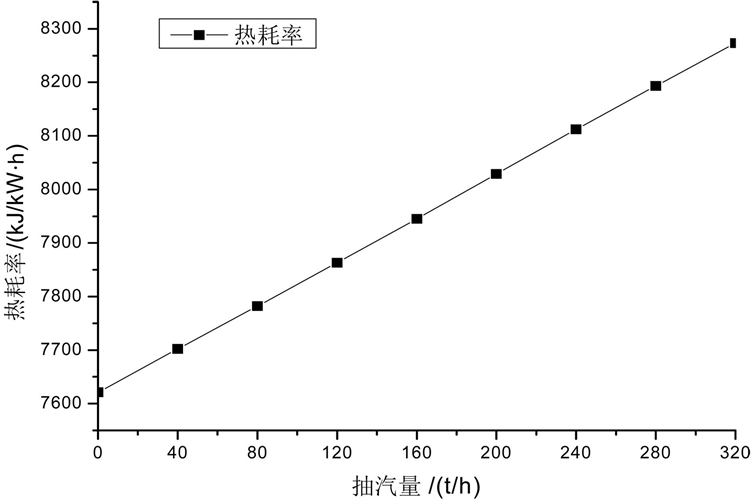Figure 10. The change of heat consumption rate of electricity generation

4.2.2. 热电厂技术指标随供热抽汽位置的变化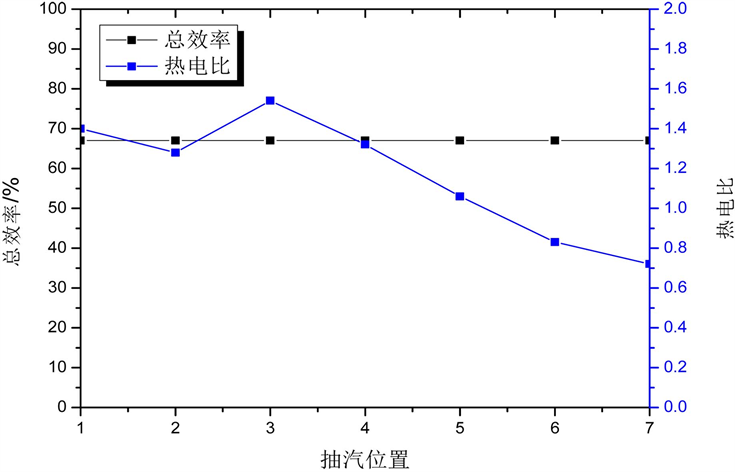Figure 11. The change of overall efficiency and ratio of heat to electricity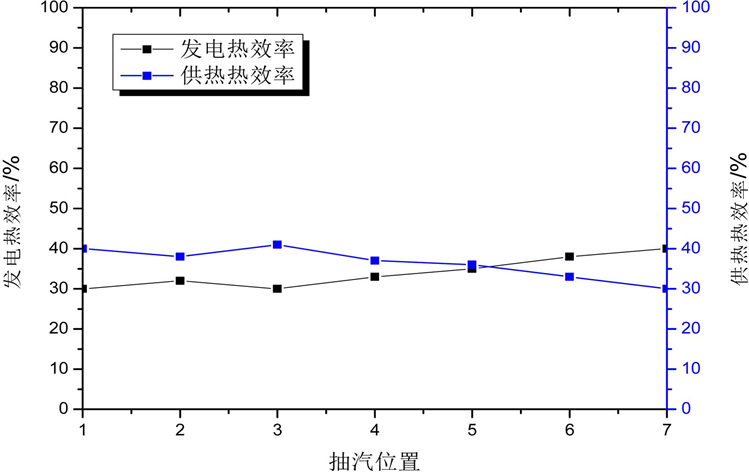Figure 12. The change of generating efficiency and heating efficiencyFigure 13. The change of heat consumption rate of electricity generation

4.3. 热电厂的㶲分析Table 9. Computed results of exergy of thermal power plant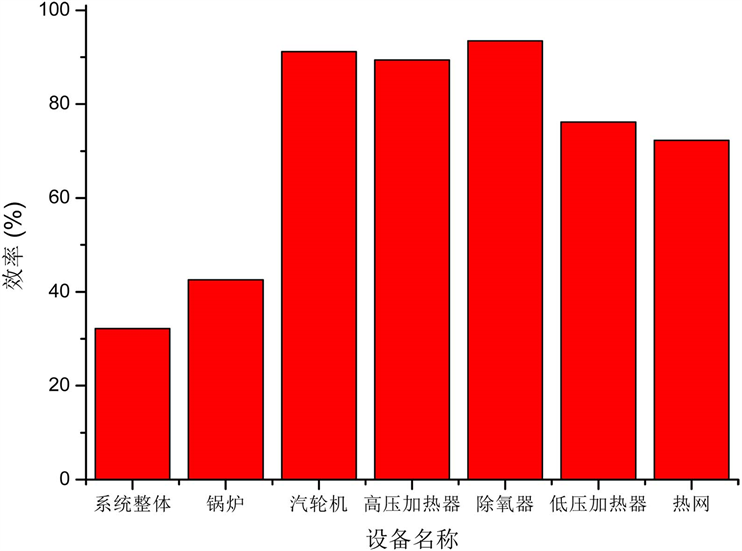Figure 14. The exergy efficiency of different equipments

5. 结论

㶲分析的计算结果表明：改造前区域供热锅炉的㶲效率只有15.35%，热电厂锅炉的㶲效率为42.6%，改造后系统的总㶲效率为32.23%，可见改造后系统的㶲效率得到明显提高。对系统各设备的㶲计算结果显示：热电厂各设备中锅炉的㶲效率明显低于其他设备，且锅炉的㶲损失占系统总㶲损失的81.2%，具有较大的节能潜力，应对锅炉设备做进一步的研究，找出㶲损失较大的环节进行改良。供热管网的㶲效率为72.3%，仅高于锅炉的㶲效率，因此在热网建设中应采取适当的节能措施，减少供热管网的㶲损失。

Energy Saving Analysis of CHP in the Heat Supply Modification[J]. 电力与能源进展, 2018, 06(01): 29-47. http://dx.doi.org/10.12677/AEPE.2018.61004

1. 1. 中国建筑科学研究院. JGJ26-1995建筑节能设计标准: 采暖居住建筑部分[S]. 北京: 中国建筑工业出版社, 2010: 91-92.

2. 2. 徐逢祥. 建筑节能形势与发展[J]. 供热信息, 2006(2): 45-49.

3. 3. 陈和平. 我国热电联产概况与政策[J]. 区域供热, 2001(2): 45-49.

4. 4. 王振铭, 郁刚. 我国热电联产的现状、前景与建议[J]. 中国电力, 2003(9): 43-49.

5. 5. 2010年热电联产发展规划及远景发展目标[Z]. 国家发展改革委员会能局, 2002: 73-75.

6. 6. 武学素. 热电联产[M]. 西安: 西安交通大学出版社, 1988: 117-121.

7. 7. 杨项劲, 由世俊. 单纯供热负荷下热电联产与热电分产的能耗分析比较[J]. 煤气与热力, 2003(2): 68-72.

8. 8. 李维仲. 锅炉热力计算与㶲分析[J]. 大连理工大学学报, 2006(4): 451-455.